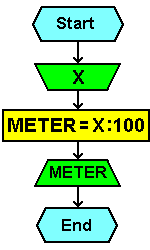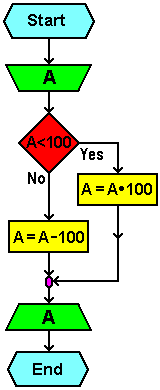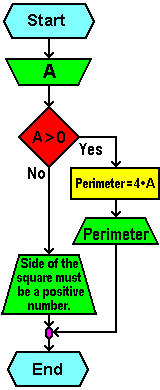## Example IVWrite an algorithm and flowchart for converting length expressed in units of measure 'centimeter' in the measuring unit 'meter'. Specifies the number of measuring in centimeters, and should determine how it meters.

Algorithm:

```start
-----------------------------------------------------
Input X
METER = X / 100
Print METER
-----------------------------------------------------
end
```
 Flowchart: Program code:``` CLS CLEAR INPUT X met = X / 100 PRINT met END ```## Example V

Write an algorithm and flowchart which a given number A increased 100 times if A is less than 100, otherwise A is decreased by the 100. Print this result.

Algorithm:

```start
-----------------------------------------------------
Input A
If A < 100
Then A = A × 100
Else A = A - 100
Print A
-----------------------------------------------------
end
```
 Flowchart: Program code:``` CLS CLEAR INPUT A IF A < 100 THEN A = A * 100 ELSE A = A - 100 END IF PRINT A END ```## Example VI

Write an algorithm and flowchart for calculating the perimeter of the square, if the default length of the side of the square A. Before computation to check whether the side of the square is greater than 0, and if not print a message: 'Side of the square must be a positive number.'

Algorithm:

```start
-----------------------------------------------------------
Input A
If A > 0
Then PERIMETER = 4 × A, print PERIMETER
Else print 'Side of the square must be a positive number.'
-----------------------------------------------------------
end
```
 Flowchart: Program code:``` CLS CLEAR INPUT A IF A > 0 THEN per = A * 4 PRINT "Perimeter=" per ELSE PRINT "Side of square must be" PRINT "a positive number." END IF END ```Citing of this page: Radic, Drago. " IT - Informatics Alphabet " Split-Croatia. {Date of access}. . Copyright © by Drago Radic. All rights reserved. | Disclaimer